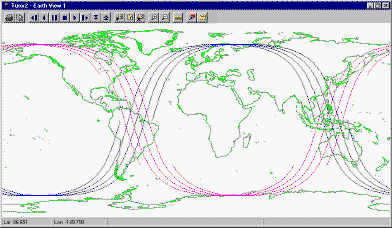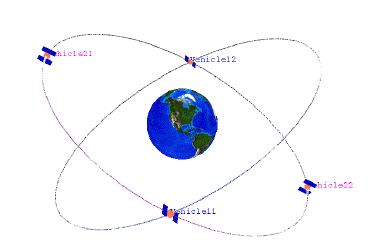# Walker Tool

The Walker Tool makes it easy to generate a Walker constellation using the Two Body, J2, J4, or SGP4 orbit propagators. First, define a satellite with the characteristics and orbit you need and then open the Walker tool by highlighting the satellite in the Object Browser and selecting Walker... from the Satellite menu.

The original satellite that you defined is referred to as the seed satellite, while the satellites generated using the Walker tool are referred to as the children.

If the seed satellite has sub-objects such as sensors, the sub-objects are also created for each of the child satellites.

A Walker constellation consists of a group of satellites (t) that are in circular orbits and have the same period and inclination. The pattern of the constellation consists of evenly spaced satellites (s) in each of the orbital planes (p) specified so that t=sp. The ascending nodes of the orbital planes are also evenly spaced over a range of right ascensions (RAAN).

If you want a grouping of sensors on the satellites in the constellation, create them on the seed satellite and they are copied onto members of the constellation during the creation of the constellation.

The way in which spacing between the ascending nodes that define the orbital planes is calculated depends on the Type of Walker constellation you choose. In addition to specifying the number of satellites in each plane, you must also specify the location of the first satellite in each plane relative to the first satellite in adjacent planes. The way to specify the position of the first satellite depends on the type of Walker constellation you choose.

## Defining a Walker Constellation

Walker constellations are based on a simple design strategy for distributing the satellites in a constellation. There are two main variants of Walker constellations: Walker Delta constellations and Walker Star constellations. The two variants differ in the distribution of the ascending nodes between the planes of the constellation. For a Walker Delta constellation type, the ascending nodes of the planes are distributed over the full range of 360 degrees, while in the Star configuration, the ascending nodes are distributed over a 180 degree span. Walker constellations are often identified using the following shorthand notation,

i:t/p/f

where i is the inclination of the orbital planes, t is the total number of satellites in the constellation, p is the number of planes and f is an inter-plane phasing designation. The relative along-track position of two satellites in adjacent planes is determined by the phase parameter (f) where f is an integer from 0 to p-1. The value of f represents the number of slots of angular measure (360 degrees/t) by which the more easterly satellite leads the more westerly satellite.

## Walker Constellation Options

The options that are available are dependent on the type of constellation selected.

Option Description
Type Select one of the following types:
• Delta. Delta configurations have orbit planes distributed evenly over a span of 360 degrees in right ascension. Requires an integer value of f for inter-plane phasing.

• Star. Star configurations have orbit planes distributed over a span of 180 degrees. Requires an integer value of f for inter-plane phasing.
• Custom. A Custom configuration allows for explicit input of the span over which ascending nodes should be distributed and allows for the explicit specification of inter-plane phasing in terms of a true anomaly offset.
Number of Sats per Plane The number of satellites to be generated in each plane of the constellation(s). The number of satellites are entered as either the total number of satellites in the constellation or the number of satellites per plane.
Total Number of Satellites Select as an alternative to Number of Sats per Plane.
Number of Planes The number of orbital planes to be used in the constellation (p).
Inter Plane Spacing

The number of "slots" between the first vehicle in adjacent planes (f). Interplane spacing can be, at most, one less than the number of orbital planes to be used in the constellation (p).

Replaced by True Anomaly Phasing for Custom configurations.

True Anomaly Phasing

In-track spacing between the first satellite in adjacent planes, entered in degrees.

Replaced by Inter Plane Spacing for Delta and Star configurations.

The angle to be divided by the number of planes to yield the difference in right ascension of the ascending node between adjacent planes.

Fixed at 360 degrees for Delta configurations and 180 degrees for Star configurations.

Replaced by RAAN Increment for Custom configurations.

Plane 1 will use the RAAN value defined by the reference satellite. The RAAN for subsequent planes is computed by adding (RAAN Spread / Number of Planes) to the RAAN of the prior plane.

The RAAN spread is important for small constellations in preventing undesirable plane orientations. For example, if there were two orbit planes inclined at 90 degrees, a RAAN spread of 180 degrees would result in two perpendicular orbit planes while a RAAN spread of 360 degrees would result in all the satellites being in the same plane and in danger of head-on collisions.

RAAN Increment Spacing of RAAN between orbital planes, entered in degrees. Replaced by RAAN Spread for Delta and Star configurations.
Color by Plane Select to set the display color of the satellites in the 2D Graphics window by their planes; all of the satellites in the same plane are displayed in the same color, and each plane is assigned a different color from the other planes. If this option is not selected, all child satellites will inherit the display color of the seed satellite.
Create unique names for sub-objects Select to have unique names assigned to all of the sub-objects of the satellites. If this option is not selected, all sub-objects for all of the child satellites will inherit the sub-object names given for the seed satellite when the constellation is created.
Create Constellation Select to have STK automatically create a Constellation object that includes all the satellites in the Walker constellation. Enter the constellation's name in the text box.

When a Walker constellation is created, the original (seed) satellite is duplicated as part of the constellation. The new satellites are considered as children of the seed. If you open a Walker window for a child satellite, all fields are grayed out. In addition, the Number of Planes field and Number of Satellites per Plane field shows the plane number and satellite number for the selected child satellite. Each child has the same base name as the seed satellite plus two numbers: the first number identifies the plane in which the satellite resides and the second identifies the satellite's position in the plane.

For instance, if you defined a Walker constellation as having 2 Planes, 2 Satellites per Plane, an Interplane Spacing of 1 and a RAAN Spread of 360 degrees, the 2D Graphics window would look similar to that in the figure.The 2D Graphics window showing a Walker seed satellite and its children (two planes each with two satellites)

The next figure more clearly shows the configuration and spacing of the satellites.Walker constellation clearly illustrating the satellite configuration

The table describes the spacing between satellites in more detail.

Satellite spacing for sample Walker satellites

Satellites RAAN Argument of Perigee True Anomaly
Seed Satellite 0 degrees 0 degrees 0 degrees
Satellite11 0 degrees 0 degrees 0 degrees
Satellite12 0 degrees 0 degrees 180 degrees
Satellite21 180 degrees 0 degrees 90 degrees
Satellite22 180 degrees 0 degrees 270 degrees